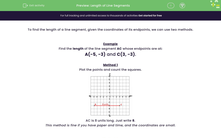# Find the Length of Line Segments

In this worksheet, students will state the length of a vertical or horizontal line segment when they are given the coordinates of its endpoints.Key stage:  KS 3

Curriculum topic:   Algebra

Curriculum subtopic:   Use Coordinates in All Four Quadrants

Popular topics:   Algebra worksheets, Year 7 Algebra worksheets

Difficulty level:#### Worksheet Overview

To find the length of a line segment, given the coordinates of its endpoints, we can use two methods.

Example

Find the length of the line segment AC whose endpoints are at:

A(-5, -3) and C(3, -3).

Method 1

Plot the points and count the squares.

This is a good method if you have time to do it and paper and pencil to hand!AC is 8 units long. Just write 8

Method 2

If you don't have the time to plot the coordinates, this method is the best!

Subtract the relevant coordinates.

The x-coordinates of the endpoints of vertical lines will be the same.

The y-coordinates of the endpoints of horizontal lines will be the same.

A is at (-5, -3) and C is at (3, -3).

The line AC is horizontal because the y-coordinates are both -3.

Subtract the x-coordinates:  -5 - 3 = -8 or 3 - -5 = 8.

Ignore the sign:  -8 or 8 means a length of 8 units. Just write 8.

For vertical lines, we would subtract the y-coordinates.

Let's give it a try in some questions now.### What is EdPlace?

We're your National Curriculum aligned online education content provider helping each child succeed in English, maths and science from year 1 to GCSE. With an EdPlace account you’ll be able to track and measure progress, helping each child achieve their best. We build confidence and attainment by personalising each child’s learning at a level that suits them.

Get started